# Sudoku Solver using Image Processing and Neural Networks

Can Neural Networks and Image Processing be able to solve sudoku puzzles?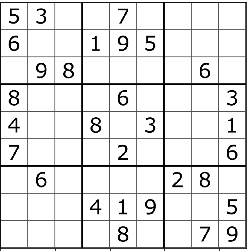Image from Wikipedia

I love solving sudoku puzzles. After reading articles in the local newspaper, I’m solving the sudoku and crossword puzzle. It is a good brain exercise to start the morning. After learning some lessons on image processing, I just thought, can I use what I learned to automatically solve these from scanned images? My teammates and I came up with a simple algorithm to solve this. Many thanks to Tal, Lyon, and Aids for helping me creating this.

Overall Process

The process of making this are the following:

1. Binarization of Images

In my previous post, we were able to perform binarization and extracting data from images. You may visit this post for more details about the first two steps. https://kdtabongds.medium.com/extracting-data-from-photos-using-image-processing-techniques-4b0f665825d1.

The code below is tailor-fit for a sudoku puzzle.

`import matplotlib.pyplot as pltimport numpy as npimport pandas as pdfrom skimage.io import imread, imshowfrom skimage.measure import label, regionprops, regionprops_tablefrom skimage.color import rgb2grayfrom skimage.transform import resizesudoku_image = imread('sudoku.png')sudoku_image = resize(sudoku_image, (900, 900), anti_aliasing=True)def bounding_box(image):        """    Returns an image with the corresponding bounding box plotted.        """    #Binarization    bin_image = rgb2gray(image) > 0.3    label_im = label(bin_image, background=1)            #Region Props    regions = regionprops(label_im)    fig, ax = plt.subplots(figsize=(5,5))    count = 0    for props in regions:        minr, minc, maxr, maxc = props.bbox        area = (maxr - minr)*(maxc - minc)        #Filtering Box by Area        if area > 500 and area < 4000:            bx = (minc, maxc, maxc, minc, minc)            by = (minr, minr, maxr, maxr, minr)            ax.plot(bx, by, '-r', linewidth=2)            count += 1         #Counting the number of fruits           ax.set_title("Number of Box : {}".format(count))    ax.imshow(image, alpha = 0.5)bounding_box(sudoku_image)`

This code will add bounding boxes to the detected digits in the image. The coordinates of the boxes can be as slicing index to produce the numbers are images. These images are then read by the neural network to predict the digits.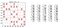Images from Author

Apply Convolutional Neural Network to predict the digits.

There are different datasets available to train a neural network in digit recognition. There are also OCR tools to read numbers from images. In this post, we used the MNIST handwritten dataset in predicting the numbers from images. Sounds ironic since the sudoku digits are not handwritten. We chose this dataset anyway because it has the largest dataset and produces the highest accuracy on test data (the test data refers to recognizing the sudoku digits)

There are numerous sources on how to train a neural network using the MNIST dataset. This is the most useful reference for me: https://machinelearningmastery.com/how-to-develop-a-convolutional-neural-network-from-scratch-for-mnist-handwritten-digit-classification/

You can use the CNN model to predict the digits. Take note that you need to reshape first the image before predicting the image. You may do this by using this code:

`from skimage.transform import resizeimport numpy as npimage = resize(image, (28, 28), anti_aliasing=True)image = image.reshape(1,28,28,1)`

As you can see, we can use a neural network to predict the numbers represented by the images.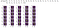Image from Author

We can then map out the location of these numbers by using the coordinates of the bounding boxes. In summary, the process converts the sudoku image into a 9x9 array. This array shall be used as input to py-sudoku, a library for solving a sudoku puzzle.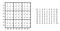Image from Author

Apply py-sudoku in solving the puzzle

Applying py-sudoku is straightforward. You just need to input a 9x9 array into the program, then the library will give you the solution. You need to install this on your machine via pip install.

`from sudoku import Sudokuboard = [[5, 3, 0, 0, 7, 0, 0, 0, 0], [6, 0, 0, 1, 9, 5, 0, 0, 0], [0, 9, 8, 0, 0, 0, 0, 6, 0], [8, 0, 0, 0, 6, 0, 0, 0, 3], [4, 0, 0, 8, 0, 3, 0, 0, 1], [7, 0, 0, 0, 2, 0, 0, 0, 6], [0, 6, 0, 0, 0, 0, 2, 8, 0], [0, 0, 0, 4, 1, 9, 0, 0, 5], [0, 0, 0, 0, 8, 0, 0, 7, 9]]puzzle = Sudoku(3, 3, board=board)puzzle.solve().show()`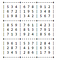Image from Author

There are many things that can be improved from this approach. For instance, we can use homography to read images on non orthogonal views of the images, balancing techniques on dark captured images, and filters on images with noise. Moreover, we can use opencv and other similar libraries to have a real time sudoku solver on a camera. That is for later. Thanks for reading this post.

Learning more about Image Processing using Python

## More from Kris Tabong

Learning more about Image Processing using Python